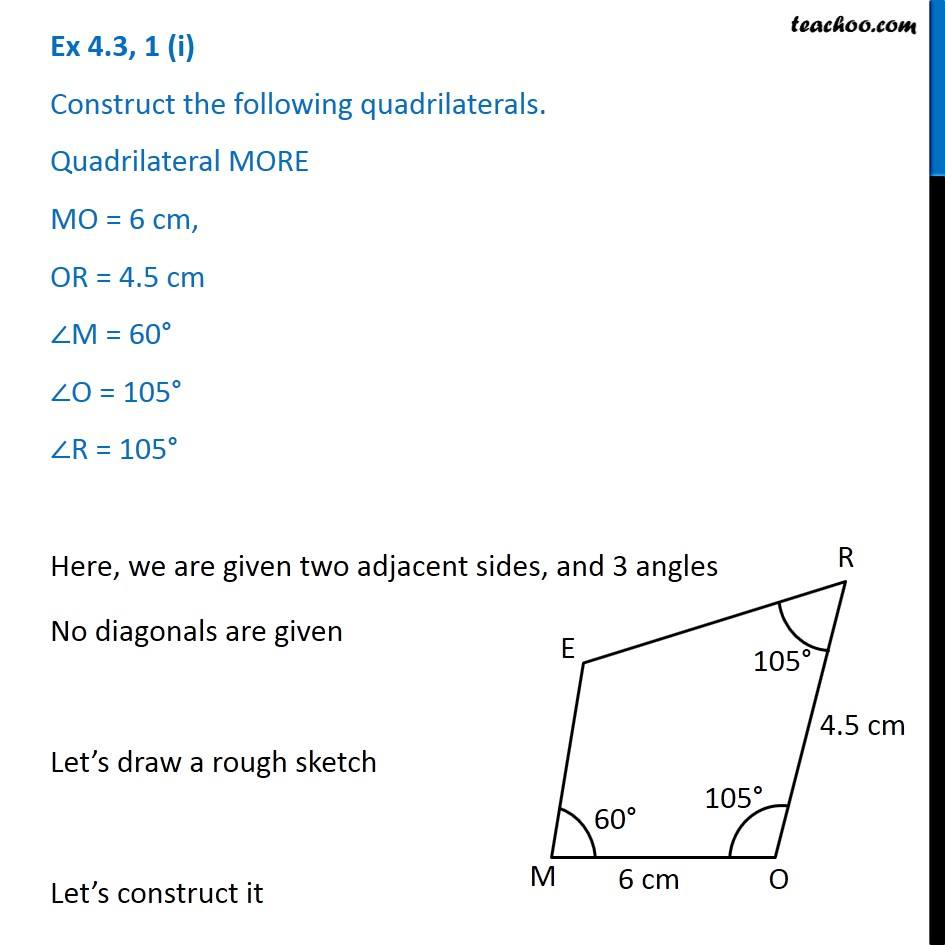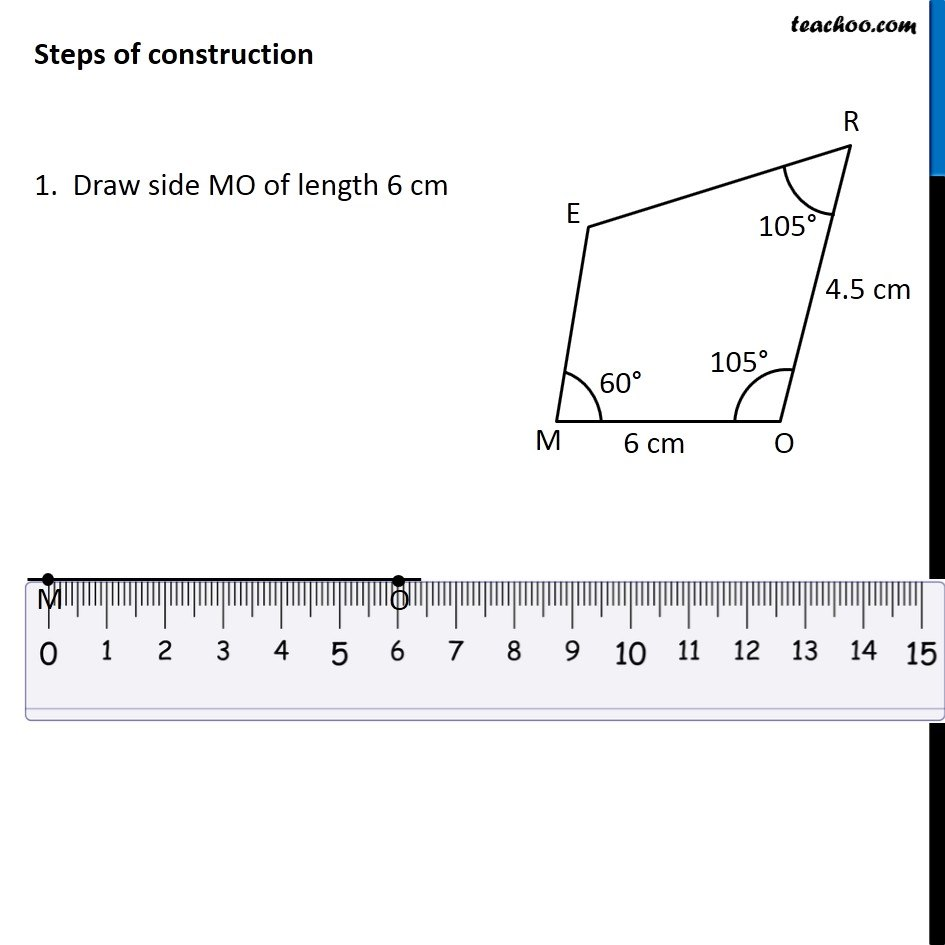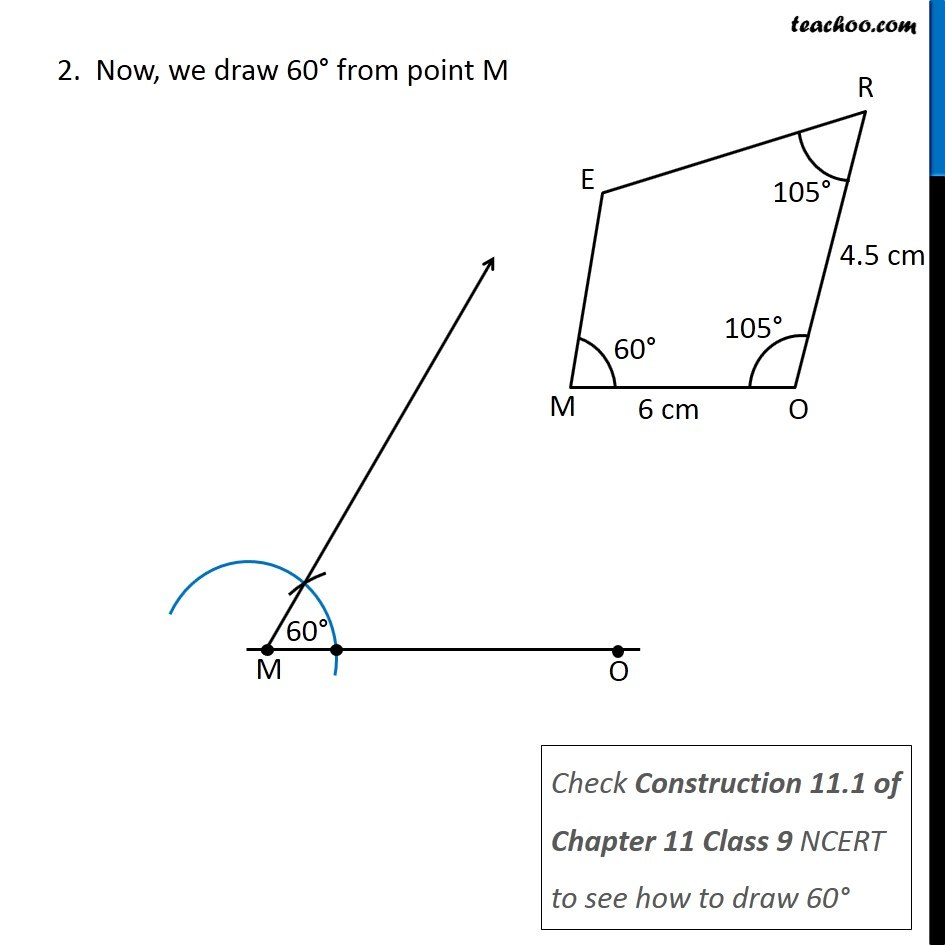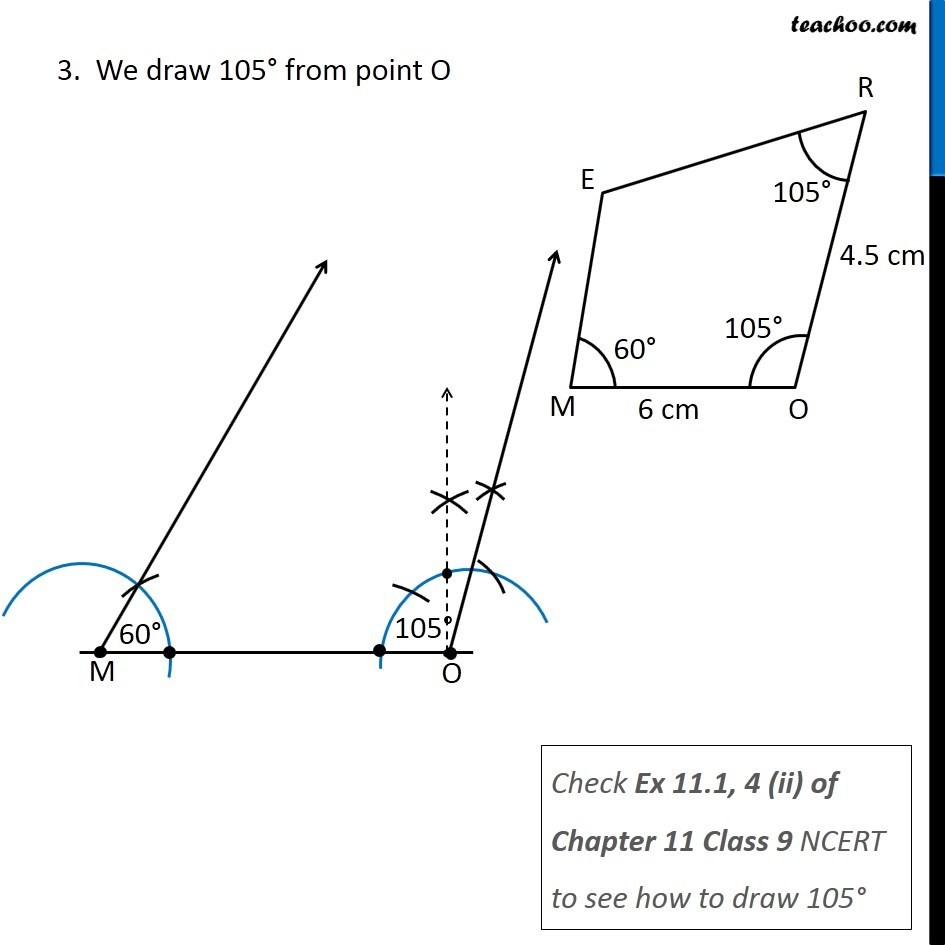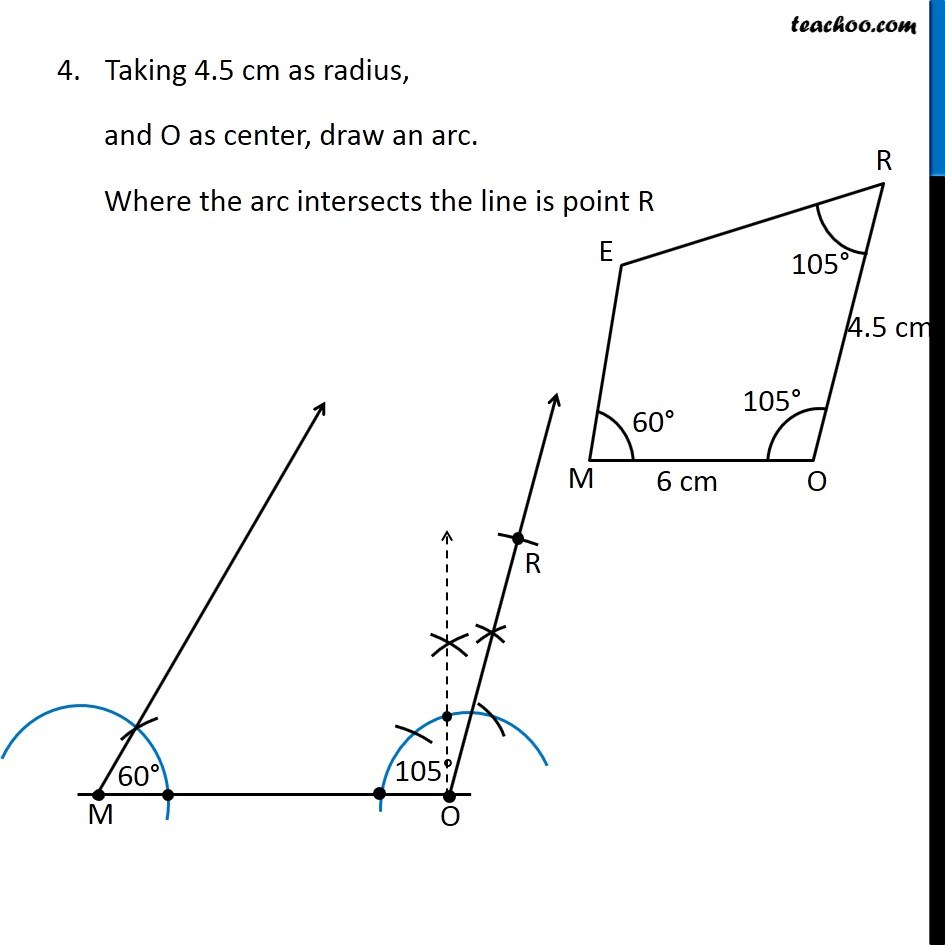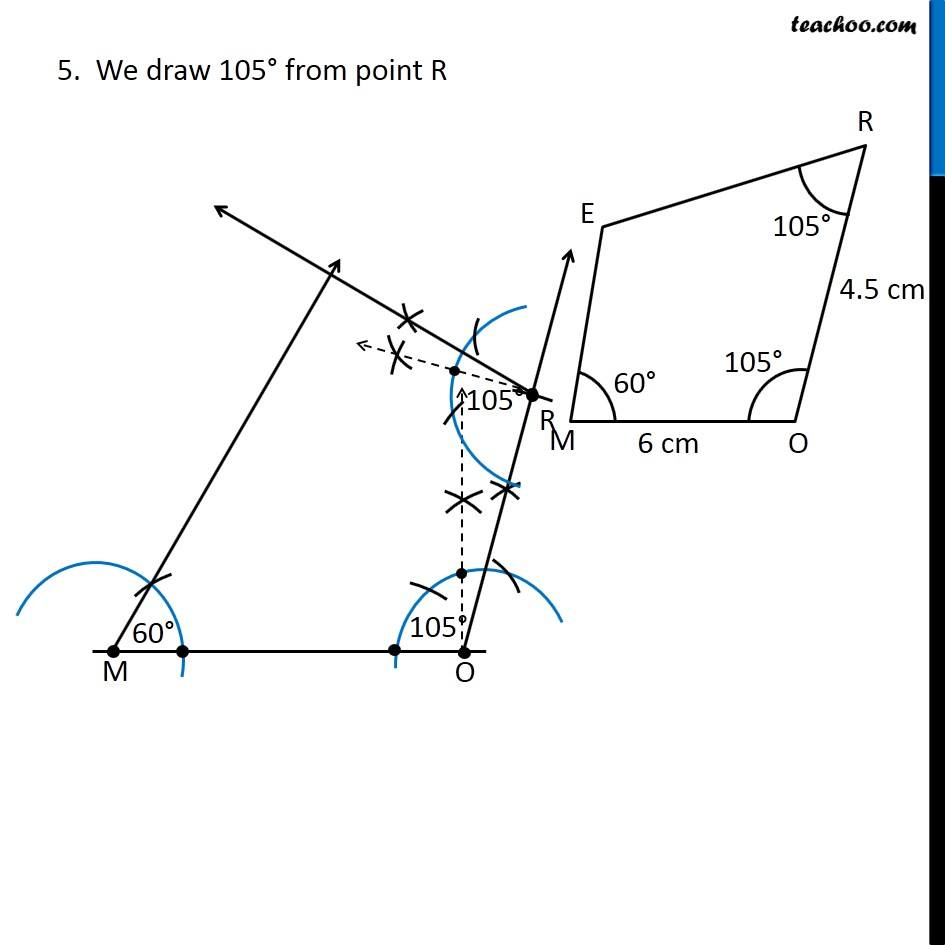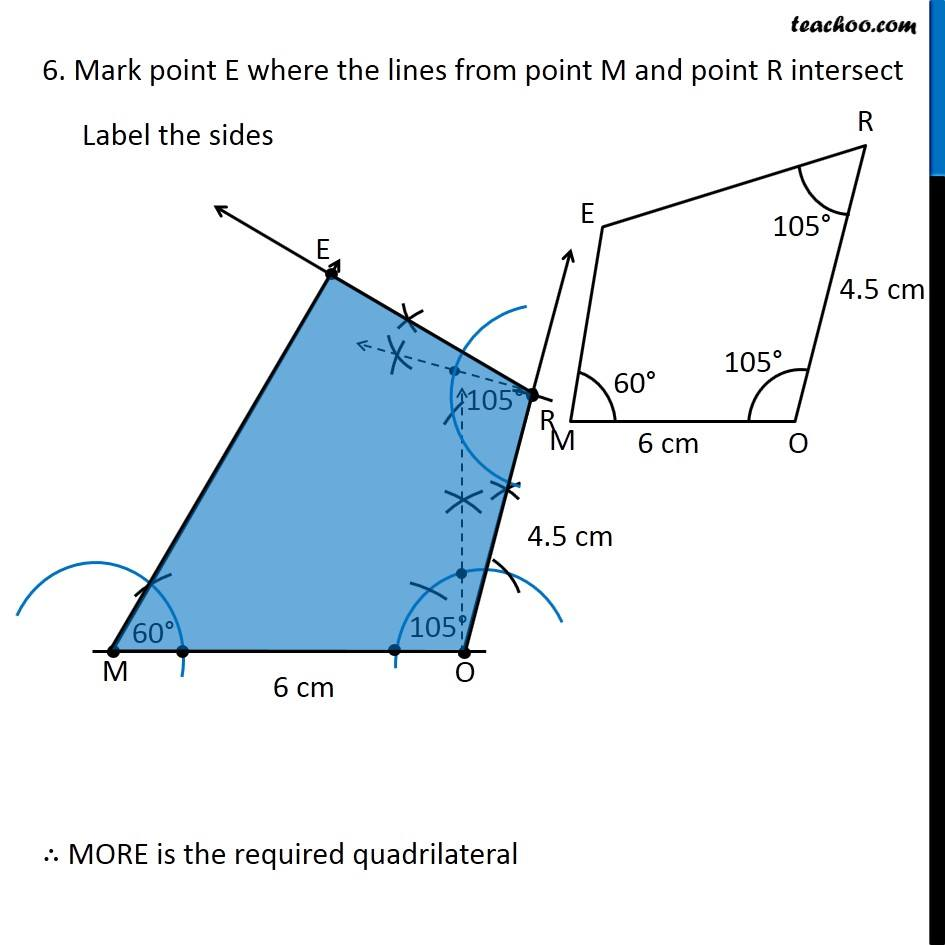for refrence

Learn in your speed, with individual attention - Teachoo Maths 1-on-1 Class

### Transcript

Question 1 (i) Construct the following quadrilaterals. Quadrilateral MORE MO = 6 cm, OR = 4.5 cm ∠M = 60° ∠O = 105° ∠R = 105° Here, we are given two adjacent sides, and 3 angles No diagonals are given Let’s draw a rough sketch Let’s construct it Steps of construction 1. Draw side MO of length 6 cm 2. Now, we draw 60° from point M Check Construction 11.1 of Chapter 11 Class 9 NCERT to see how to draw 60° 3. We draw 105° from point O Check Ex 11.1, 4 (ii) of Chapter 11 Class 9 NCERT to see how to draw 105° Taking 4.5 cm as radius, and O as center, draw an arc. Where the arc intersects the line is point R 5. We draw 105° from point R 6. Mark point E where the lines from point M and point R intersect Label the sides ∴ MORE is the required quadrilateral